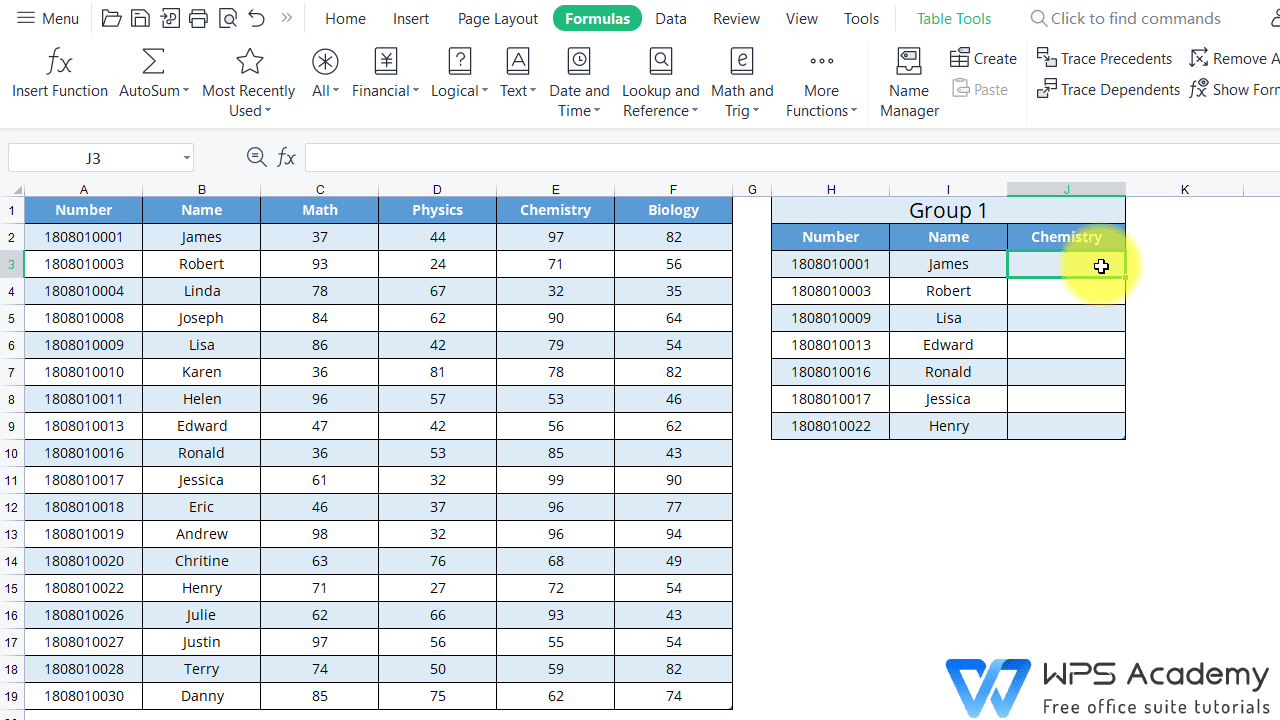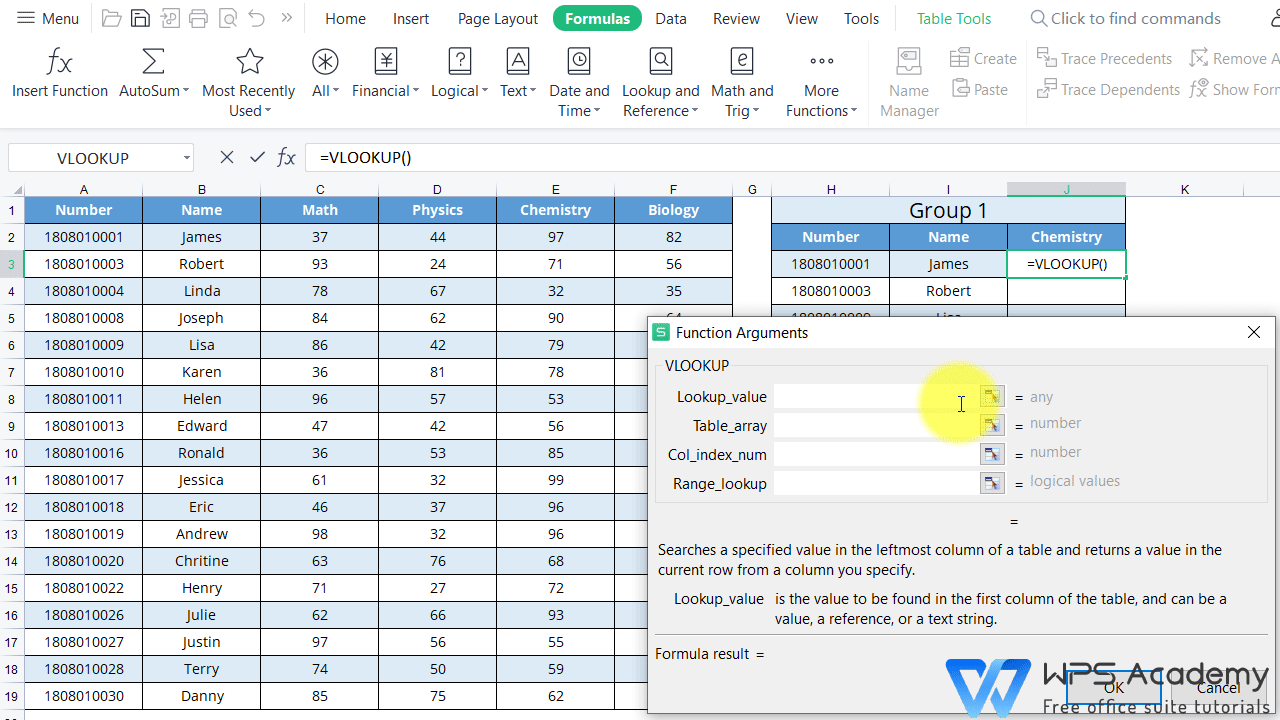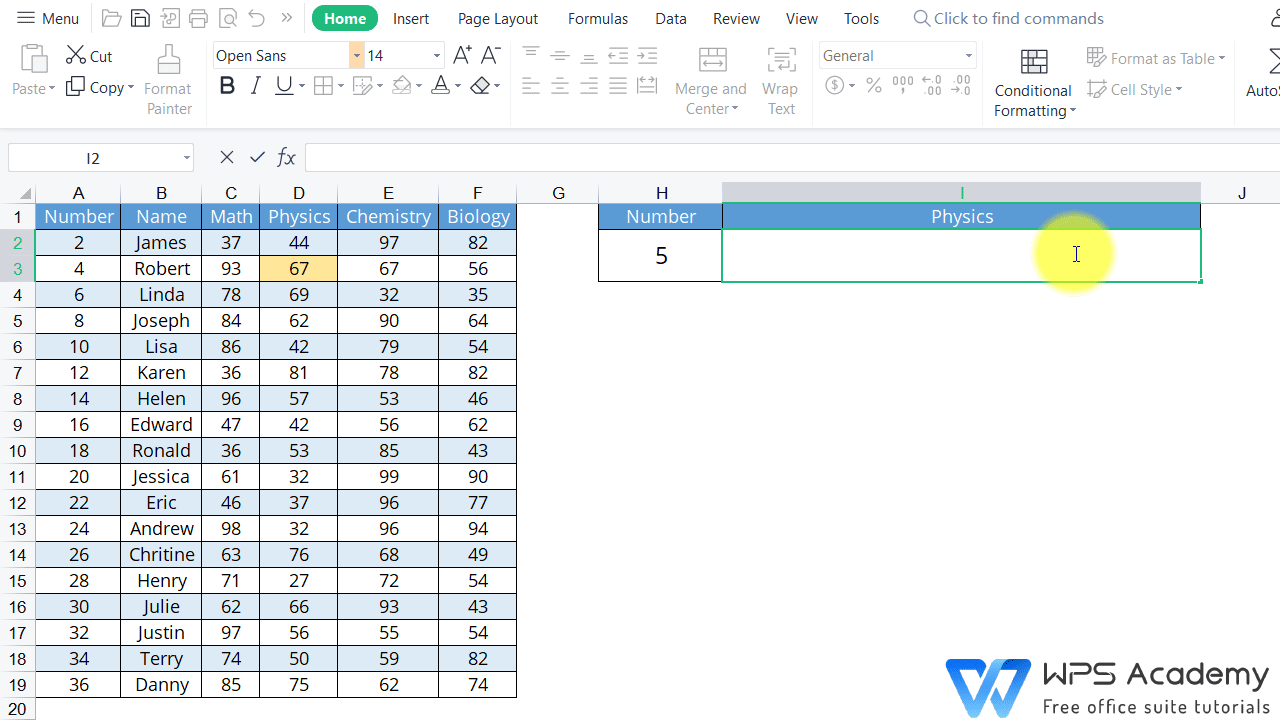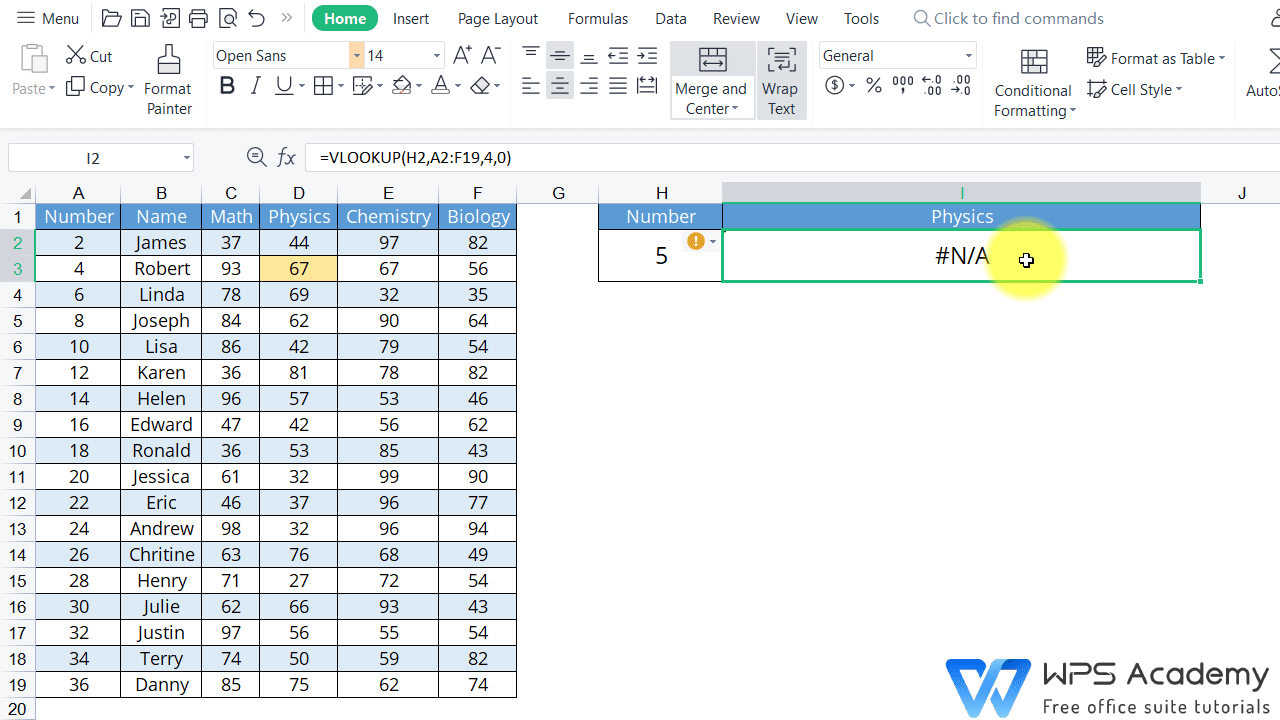# How to use the VLOOKUP function

Uploaded time: July 22, 2022 Difficulty Beginner

A free Office suite fully compatible with Microsoft Office

How to use the VLOOKUP function

# How to use the VLOOKUP function

The clever use of VLOOKUP function can help us quickly find the relevant results. It is usually used to check and match the data between multiple tables.

Take this table as an example. In the table on the right, we know the Number and Name of the Group1 members, so we are looking for the Chemistry scores of the members.

1. Click cell J3, and click Insert Function.

2.Click Lookup and Reference in Category, and choose VLOOKUP function.

3.In the popup dialog box, we can see that there are 4 parameters, namely Lookup_value, Table_array, Col_index_num and Range_lookup.Lookup_value is the value to be found. We need to look up the Number 1808010001 in the table on the right to find its corresponding scores of various subjects. So, we should enter H3 here.

Table_array refers to the area where the data is to be found. At this time, the scores of all subjects of the Group 1 students we are looking for are in the left summary table. So we refer to the data table A2:F19 and click Shift+F4 to add an absolute reference.

Col_index_num refers to the number of columns in the selected area. In this case, the chemistry scores that we are looking for are in the fifth column of the left table. Here we enter 5.

Range_lookup: In this option, we can choose Find the closest match or Find an exact match. Entering FALSE or 0 indicates the exact match, and entering True or 1 indicates the closest match. Here, we enter 0 to indicate an exact match.

Finally, click OK to get the results and fill in the table.VLOOKUP function still has several points that require our special attention.

1.This function can only match data from columns to the right of the first column in the table.

Let's go back to the case, and the Employee Number column is on the left of the Name column. So, it's impossible to find the Employee Number by Name. However, we can use Employee Number to find a Name .

2.The difference between Find the closest match and Find an exact match: The exact match represents a full search. If the same value is not found, we return the error value #N/A. The closest match first finds the same value and then finds a very close value if the same value cannot be found. If we cannot find a close value, we will also return the error value #N/A.

As shown in the video, suppose what we are looking for is the score in physics of a student whose school number is 5, but the student's information is not available in the referenced search area.If we choose the closest match, what we return is the physics score of the student with the number 4, which is 67. Because number 5 is closest to number 4.The following is the formula of the VLOOKUP function and the specific meaning of each parameter:

Formula: =VLOOKUP(lookup_value,Table_array,Col_index_num,Range_lookup)

Lookup_value: What you want to look up.

Table_array: Where you want to look for it.

Col_index_num: the column number in the range containing the value to return.

Range_lookup: return an Approximate or Exact match – indicated as 1/TRUE, or 0/FALSE.

In actual working and realistic life, we can also use the VLOOKUP function for table splitting and merging, multi-condition search and so on. For more tips on using VLOOKUP function, you're welcome to WPS Academy.